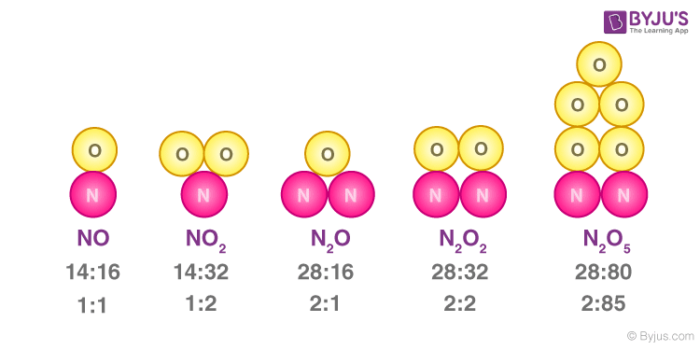# Law Of Constant Proportions

## What is the Law of Constant Proportions?

The law of constant proportions states that chemical compounds are made up of elements that are present in a fixed ratio by mass. This implies that any pure sample of a compound, no matter the source, will always consist of the same elements that are present in the same ratio by mass. For example, pure water will always contain hydrogen and oxygen in a fixed mass ratio (a gram of water consists of approximately 0.11 grams of hydrogen and 0.88 grams of oxygen, the ratio is 1:8).

The law of constant proportions is often referred to as Proust’s law or as the law of definite proportions. An illustration describing the mass ratio of elements in a few compounds is provided below. The ratio of the number of atoms of each element is provided below the mass ratio. For example, in a nitrogen dioxide (NO2) molecule, the ratio of the number of nitrogen and oxygen atoms is 1:2 but the mass ratio is 14:32 (or 7:16).In the year 1794, the French chemist Joseph Proust formulated the law of constant proportions from his work on sulphides, metallic oxides, and sulfates. This law was met with a lot of opposition in the scientific community in the 18th century. The introduction of Dalton’s atomic theory favoured this law and a relationship between these two concepts was established by the Swedish chemist Jacob Berzelius in the year 1811.

## What are the Exceptions to the Law of Constant Proportions?

Despite being a building block in the development of chemistry, the law of constant proportions does not hold true for all chemical compounds. Some exceptions to this law are listed below.

• Some non-stoichiometric compounds have varying compositions of elements between samples. These compounds obey the law of multiple proportions instead.
• One such example is wustite, an oxide of iron with the chemical formula FeO. The ratio of iron and oxygen atoms can range from 0.83:1 to 0.95:1.
• This is caused by the crystallographic vacancies in the samples caused by a disorderly arrangement of atoms.
• Various samples of a compound may vary in the isotopic composition of its constituent elements. This can lead to fluctuations in the mass ratios.
• The differences in the mass ratios between samples are very useful in the process of geochemical dating, due to the preferential concentration of isotopes in many deep Earth and crustal processes.
• This also occurs in many oceanic, atmospheric and even astronomical processes. Despite the effects being quite small, the challenges in the measurement of the effects have been overcome by modern instrumentation.
• Since natural polymers can vary in their compositions, various samples can show different mass proportions.

## Frequently Asked Questions on the Law of Constant Proportions

### What is the Statement of the Law of Definite Proportions?

The law of definite proportions, also known as the law of constant proportions, states that the individual elements that constitute a chemical compound are always present in a fixed ratio (in terms of their mass). This ratio does not depend on the source of the chemical compound or the method through which it was prepared.

### What are the Exceptions to the Law of Constant Proportions?

The ratio of elements in non-stoichiometric compounds varies from sample to sample. Therefore, these compounds are an exception to the law of constant proportions. Samples of elements that vary in their isotopic composition can also defy the law of definite proportions since the masses of two different isotopes of an element are different. Natural polymers are also known to disobey the law of constant proportions.

### Who theorized the law of definite proportions?

The law of definite proportions was first put forward by the French chemist Joseph Louis Proust in the year 1779. This is the reason why this law is also known as Proust’s law. The observations associated with this law were first made by the French chemists Antoine Lavoisier and Joseph Priestley.

### Give some examples of compounds that obey the law of definite proportions.

Water molecules feature the combinations of hydrogen and oxygen atoms in a 2:1 ratio. Since they are present in a fixed ratio of mass, water molecules obey the law of constant proportions. Another example of a chemical compound that obeys the law of constant proportions is methane. To form one methane molecule, 4 hydrogen atoms combine with 1 carbon atom.

### What is the significance of the law of definite proportions?

Although this law is easily understandable today, it was of great use in the late 18th century when chemical compounds did not have any proper definition. The law of definite proportions also contributed to the development of Dalton’s atomic theory.

### Is the law of constant proportion true?

No, for all forms of substances, the law of definite proportion is not valid. Elements with a stable isotope mixture often form a non-stoichiometric product. The role of certain elements in the crystal structure is replaced by their isotopes which induces the crystal’s internal composition to vary.

### Which postulate of Dalton’s theory was correct?

An atom’s indivisibility has been proven wrong: it is possible to further subdivide an atom into protons , neutrons and electrons. However, the smallest particle that occurs in chemical reactions is an electron. The atoms of the same product are identical in all respects, according to Dalton.